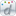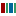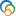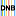The Library of Congress > Linked Data Service > LC Subject Headings (LCSH)

# Approximation algorithms

• ### Closely Matching Concepts from Other Schemes

•Algorithmes d'approximation•approximation algorithmLabel from public data source Wikidata
•Approximation algorithms•Approximationsalgorithmus• ### Sources

• found: Work cat.: Williamson, D. Lecture notes on approximation algorithms, 1998.
• found: Dictionary of algorithms and data structures, via WWW, Dec. 18, 2009(approximation algorithm -- An algorithm to solve an optimization problem that runs in polynomial time in the length of the input and outputs a solution that is guaranteed to be close to the optimal solution)
• found: Könemann, J. Approximation algorithms for minimum cost low degree subgraphs, 2003:p. 2 (Approximation algorithms are heuristic algorithms supplied with an upper bound on the worst case ratio between the value of any approximation solution and that of an optimum solution)
• found: Tan, K. On the role of partition inequalities in classical algorithms for Steiner problems in graphs, 2007:p. 2 (we resort to finding polynomial-time algorithms that efficiently solve the problems approximately, i.e., approximation algorithms; an approximation algorithm is a heuristic algorithm, which does not solve the given optimization problem exactly, but guarantees an upper bound on the worst case ratio between the value of any approximation solution and that of an optimum solution)
• found: FOLDOC, Dec. 18, 2009(approximation algorithm -- An algorithm for an optimisation problem that generates feasible but not necessarily optimal solutions; unlike "heuristic," the term "approximation algorithm" often implies some proven worst or average case bound on performance)
• found: Wikipedia WWW site, Jan. 4, 2010(Approximation algorithms; in computer science and operations research, approximation algorithms are algorithms used to find approximate solutions to optimization problems)
• ### Change Notes

• 2010-01-20: new

# Suggest terminology

The LC Linked Data Service welcomes any suggestions you might have about terminology used for a given heading or concept.

Would you like to suggest a change to this heading?

Please provide your name, email, and your suggestion so that we can begin assessing any terminology changes.

Fields denoted with an asterisk (*) are required.